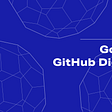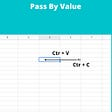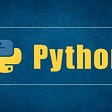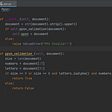# Number of Good Pairs — Day 101(Python)

After a long hiatus, I decided to start writing again as a new year resolution. I wanted to start with something easy, hence choosing the following problem.

## 1512. Number of Good Pairs

Given an array of integers `nums`, return the number of good pairs.

A pair `(i, j)` is called good if `nums[i] == nums[j]` and `i` < `j`.

Example 1:

`Input: nums = [1,2,3,1,1,3]Output: 4Explanation: There are 4 good pairs (0,3), (0,4), (3,4), (2,5) 0-indexed.`

Example 2:

`Input: nums = [1,1,1,1]Output: 6Explanation: Each pair in the array are good.`

Example 3:

`Input: nums = [1,2,3]Output: 0`

Constraints:

• `1 <= nums.length <= 100`
• `1 <= nums[i] <= 100`

This question is very similar to Two Sum. We have solved this problem before. You can find it here.

A brute force method will traverse through the list of numbers and check if the numbers match.

`class Solution:    def numIdenticalPairs(self, nums: List[int]) -> int:        output = 0        for i in range(len(nums)):            for j in range(i+1, len(nums)):                if(nums[i] == nums[j]):                    output += 1        return output`

Complexity

Time Complexity

Since we have 2 for-loops here, hence time complexity is O(N²).

Space Complexity

We are not using any extra space, and hence the space complexity will be O(1).

An optimized method will traverse through the list once, but to save time we use a dictionary to store already traversed elements. The keys will be traversed elements and the values will be the number of times we have seen that number.

`class Solution:    def numIdenticalPairs(self, nums: List[int]) -> int:        output = 0        dict_number = dict()        for n in nums:            if n in dict_number:                output += dict_number[n]                dict_number[n] += 1            else:                dict_number[n] = 1        return output`

Complexity

Time Complexity

Since we have 1 for-loop here. Hence time complexity is O(N).

Space Complexity

We are not using any extra space and hence the space complexity will be O(N).

--

--

--

## More from Annamariya Tharayil

Software Engineer. Find me @ www.linkedin.com/in/annamariya-jt

Love podcasts or audiobooks? Learn on the go with our new app.

## Golem GitHub Digest #11: Easy log collection## Sophon NFT: a Web3 diversified application ecosystem for everyone## Elixir As Your First Functional Language## Pass by Reference and Value in Swift## Daily Announcement 16th of Nov: Kujira IDO Results## Building a Next-Generation Database: Our Investment in Timescale## How to create a clean Firestore pagination with real-time updates?## Microservices: Keep the Transition Under Control## Annamariya Tharayil

Software Engineer. Find me @ www.linkedin.com/in/annamariya-jt

## How to get into coding with Pyton## Starting with the basics.## Learn Python and Get a 100k Job in 3 Months